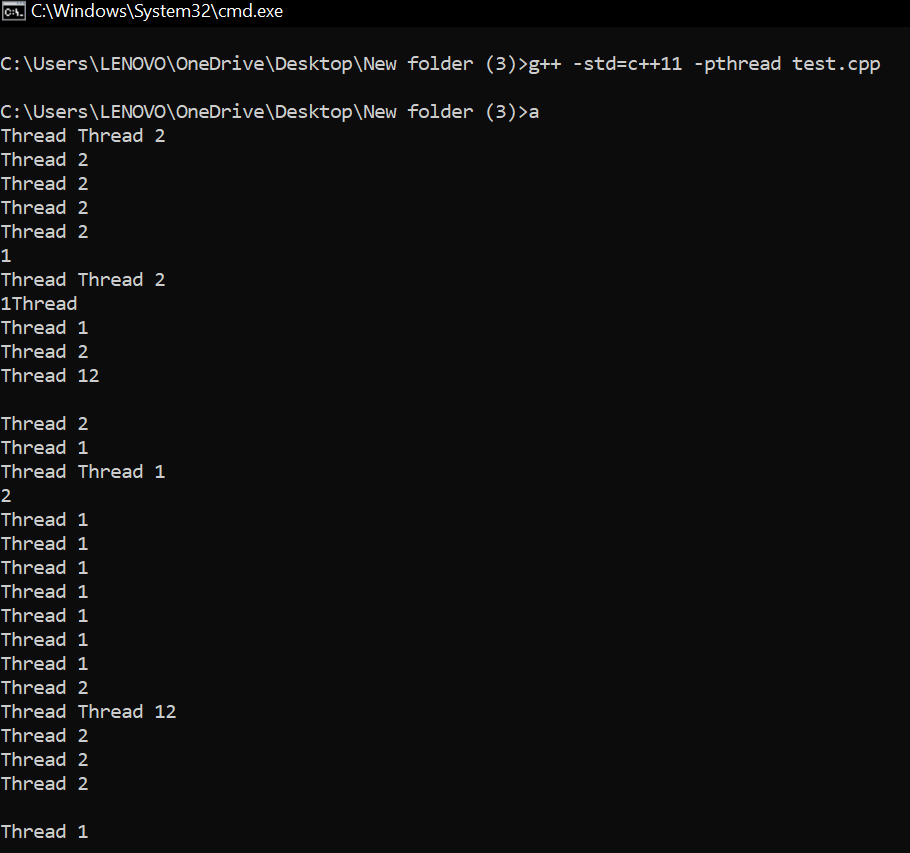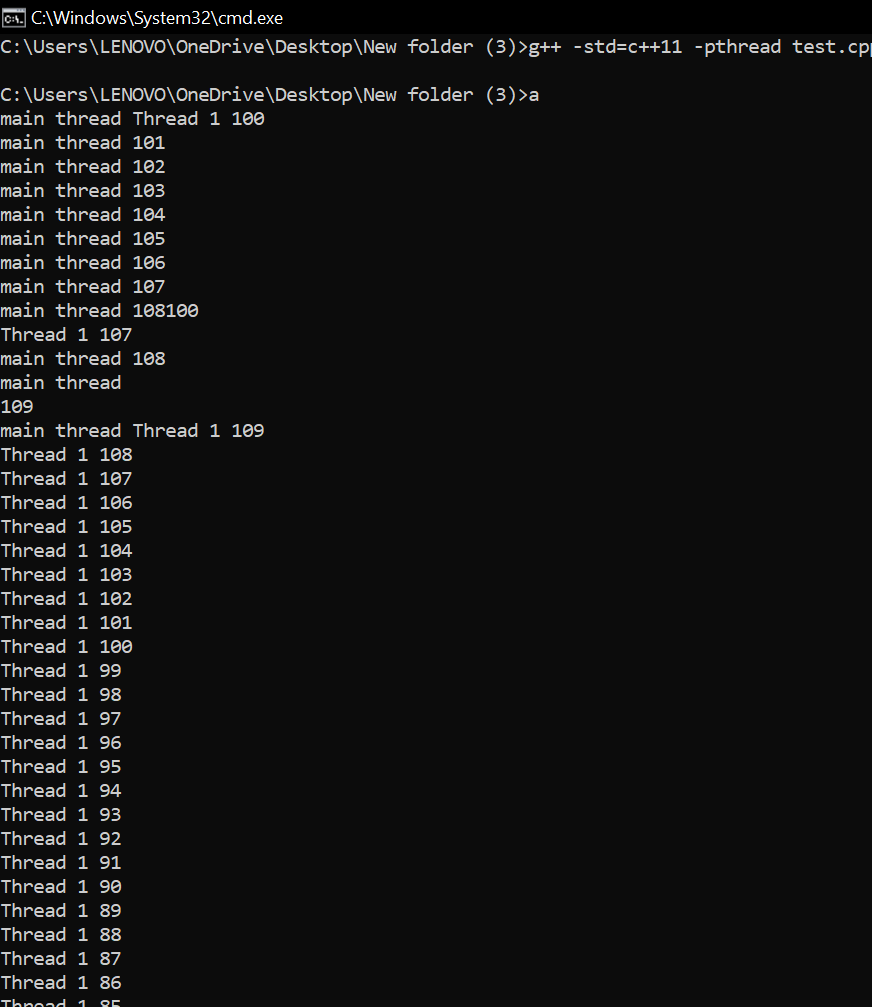# C++ Program to Show Thread Interface and Memory Consistency Errors

• Difficulty Level : Medium
• Last Updated : 29 Jul, 2022

C++ allows Multithreading by using the ‘thread’ header file. The program acts as one thread but to increase program execution time/performance we can use threads to run parts of the program concurrently. But it may lead to issues of memory consistency errors and may not give us the proper output. Threads are used to improve the performance of applications by running processes parallel to each other.

The thread may share the same resource or reference pointer. Two or more threads may refer to the same object or share some common resource and they try to update or make changes independently on shared resource data which can leave data inconsistent.

Example: In the below C++ program, two threads are used to use the same functions. First, it should run for thread 1 and then for thread 2. But to show memory consistency for sharing the same resource/function output is not consistent.

## C++14

 `// C++ program to show``// memory consistency``#include ``#include ``using` `namespace` `std;`` ` `class` `thread_obj {``public``:``  ` ` ``void` `operator()(``int` `x)`` ``{``    ``for` `(``int` `i = 0; i < 50; i++)``    ``{``      ``cout << ``"Thread "` `<< x << ``"\n"``;``    ``}`` ``}``};`` ` `// Driver code``int` `main()``{``   ``// thread 1``   ``thread` `th1(thread_obj(), 1);`` ` `   ``// thread 2``   ``thread` `th2(thread_obj(), 2);`` ` `   ``// wait for thread1 to join``   ``th1.join();`` ` `   ``// wait for thread2 to join``   ``th2.join();``   ` `   ``return` `0;``}`Output – You can see inconsistency in output (It is machine Dependent)

Example 2: In the below C++ program, an attempt will be made to access the same value from different threads as one can see memory consistency errors as both threads will run concurrently.

## C++

 `// C++ program to show memory``// consistency error``#include ``#include ``using` `namespace` `std;`` ` `int` `x = 100;`` ` `void` `thread_function() ``{``    ``for``(``int` `i = 0; i < 50; i++) ``    ``{ ``      ``x--;``      ``cout << ``"Thread 1 "` `<< ``               ``x << ``"\n"``;``    ``}``}`` ` `// Driver code``int` `main() ``{``  ``std::``thread` `t(&thread_function);``   ` `  ``for``(``int` `i = 0;i < 100; i++) ``  ``{``      ``x++;``      ``cout << ``"main thread "` `<< ``             ``x << ``"\n"``;``  ``}``       ``return` `0;``}`Output – You can see inconsistency in output (It is machine Dependent)

My Personal Notes arrow_drop_up